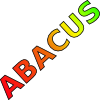#### You may also like### Consecutive Numbers

An investigation involving adding and subtracting sets of consecutive numbers. Lots to find out, lots to explore.### Tea Cups

Place the 16 different combinations of cup/saucer in this 4 by 4 arrangement so that no row or column contains more than one cup or saucer of the same colour.### Counting on Letters

The letters of the word ABACUS have been arranged in the shape of a triangle. How many different ways can you find to read the word ABACUS from this triangular pattern?

### Why do this problem?

This problem is a good one for building on the learners' ability to recognise number properties and reason about numbers. It is easy for all at the start as it only requires simple multiplication and subtraction and gives the satisfaction of finding a pattern. If the results are generalised algebraically it can prove a real challenge.

### Possible approach

You could start by posing the problem to the whole group on a computer or using a large-sized addition square.

After this learners could work in pairs on the problem so that they are able to talk through their ideas with a partner. This sheet gives two copies of the addition square which can be used for rough work.

Many learners will tend to rush on and try $2 \times 2$, $4 \times 4$ and other squares and although an interesting pattern can be found doing this, it may stop them from trying to generalise the $3 \times 3$ example. Therefore, it may be wise to stop everyone at an appropriate point and show all who need the help how the numbers can always be named $n, n + 2$ and $n + 4$. (Alternatively, they could be $n - 2$, $n$ and $n + 2$.)

At the end of the lesson learners could discuss the different patterns they have discovered and the various generalisations made.

### Key questions

How do the four numbers compare in size?
Try comparing the number in the top left corner of a square with the other three numbers.
So what happens when these numbers are multiplied in the way described?
Can you see a pattern there?
What sort of numbers are these?

### Possible extension

Learners could try to generalise, not only the results for $3 \times 3$ squares but also those for all squares on this grid. Alternatively, they could try the same procedure with rectangles rather than squares or use other grids such as multiplication squares.

### Possible support

Suggest using a calculator for the multiplication and subtraction or, alternatively, switch to Diagonal Sums which is a similar, but easier, problem.